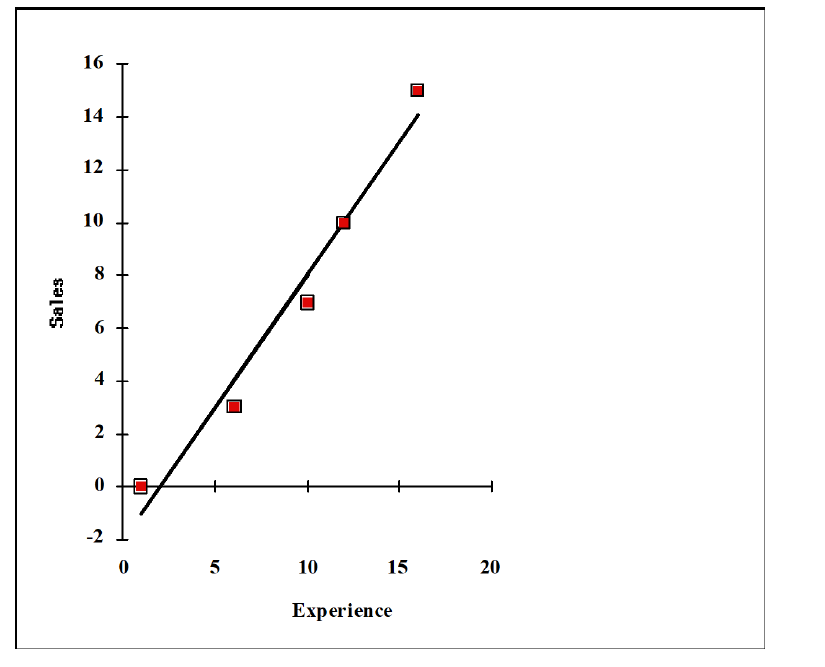top of page
Search

# MBA in 2 minutes | Lesson 4: Progressiveness of regression

Updated: Jan 8, 2021

We will be solving practical challenges through MBA concepts. No theory only applications !So today we will discuss perhaps the most business relevant topic in statistics: Simple Linear Regression. You might have heard of this term in office being used by your colleagues especially in analytics division. Frankly its pretty simple, can be even done on Microsoft excel in plain high school taught computer science level.

Step 1:

Let's first understand when and why is regression used. So we use regression to understand whether eg: a) rainfall in a city or b) number of dustbins in city market are related to the perception of city cleanliness ->if you are driving Swachh Bharat Mission.

We conduct regression analysis to conclude top 3-4 related variables for an output . Mathematically, we can put it as (y= alpha +x1+ x2+ x3.... ) where x is the independent variable and y is dependent. The coefficients of x1, x2 and x3 will determine the level of dependence of "y" on "x1, x2, x3" respectively.

For eg: You get a final regression equation, y= alpha + 5x1+ 3x2+... this imply that "y" is co-related most heavily with "x1". Thus you scientifically pump more money/effort/focus on x1 to push "y" numbers.

Step 2:

Now you plot the historical data of x and y in table on Microsoft Excel as mentioned below.Sample data that can be used

Then you draw trend line i.e. best fitting regression line. This quick step by step exercise can be found here: excel. (though we also learnt this back in high school). The trendline will look something like below graph. If you right click, you will be able to get the regression equation.Trendline

Step 3

You can also access R square value. Now what is r square value ? So always: 0< r square <1. If r square is "near" 1 it means that the regression line is a good fit to the data.

On the other hand, If the r square for a problem is “too small” or very close to 0, it indicates that the x variable may not really be related to y basically implying one shouldn't pump more money or effort in x.

Imp Note: Co-relation is not causation. Many people believe that correlation means causation. To clarify, its important to know we mean that number of dustbins are correlated to perception of cleanliness but not that number of dustbins are causing perception of cleanliness. These both are different meaning sentences and we imply former.

If you liked this post, please click that heart icon to show it. I appreciate it.

If you are new here and wish to know the story how MBA in 2 minutes was started, you can click here.

735 views1 comment
bottom of page